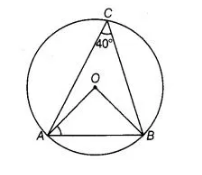# Prove the following`
Question:

In figure, $\angle A C B=40^{\circ}$. Find $\angle O A B$.Solution:

Given, $\angle A C B=40^{\circ}$

We know that, a segment subtends an angle to the circle is half the angle subtends to the centre.

$\therefore \quad \angle A O B=2 \angle A C B$

$\Rightarrow \quad \angle A C B=\frac{\angle A O B}{2}$

$40^{\circ}=\frac{1}{2} \angle A O B$

$\Rightarrow \quad \angle A O B=80^{\circ}$ $\ldots$ (i)

In $\triangle A O B$, $A O=B O$ [both are the radius of a circle]

$\Rightarrow$ $\angle O B A=\angle O A B$ ... (ii)

[angles opposite to the equal sides are equal]

We know that, the sum of all three angles in a triangle $A O B$ is $180^{\circ}$.

$\therefore$ $\angle A O B+\angle O B A+\angle O A B=180^{\circ}$

$\Rightarrow$ $80^{\circ}+\angle O A B+\angle O A B=180^{\circ}$ [from Eqs. (i) and (ii)]

$\Rightarrow \quad 2 \angle O A B=180^{\circ}-80^{\circ}$

$\therefore$ $\angle O A B=\frac{100^{\circ}}{2}=50^{\circ}$